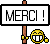[Livre] Introduction to the Finite Element Method and Implementation with MATLAB®
Introduction to the Finite Element Method and Implementation with MATLAB®

Connecting theory with numerical techniques using MATLAB®, this practical textbook equips students with the tools required to solve finite element problems. This hands-on guide covers a wide range of engineering problems through nine well-structured chapters including solid mechanics, heat transfer and fluid dynamics; equilibrium, steady state and transient; and 1-D, 2-D and 3-D problems. Engineering problems are discussed using case study examples, which are solved using a systematic approach, both by examining the steps manually and by implementing a complete MATLAB®code. This topical coverage is supplemented by discourse on meshing with a detailed explanation and implementation of 2-D meshing algorithms. Introducing theory and numerical techniques alongside comprehensive examples this text increases engagement and provides students with the confidence needed to implement their own computer codes to solve given problems.

Drawing upon years of practical experience and using numerous examples and illustrative applications, Gang Li provides:

15 engineering problems on elasticity, heat transfer, fluid flow, and mesh creation, fully worked and described in step-by-step detail
over 110 end-of-chapter problems for students to practice and build confidence
M-files for all numerical examples and exercise problems, which can be accessed and downloaded from the textbook's online resource page.

Dr. Gang Li is a professor and D. W. Reynolds Emerging Scholar of Mechanical Engineering at Clemson University. He was an awardee of the National Science Foundation Early Career Award. He is an associate editor of the Journal of Computational Electronics and serves on the ASME Committee on Computing in Applied Mechanics. Dr. Li's scholarly articles on computational mechanics and finite element method frequently appear in leading journals.

[Lire la suite]